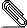# [NTG-context] Bug in latest beta: behavior of \text in StepCharts

Hi Hans,

It seems that with the latest beta there is a bug in the way how the command
\text is handled in step charts (plesae see the attached PDF).
This bug is absent from mkii, and absent from previous versions of mkiv (up to
a weak ago, I believe).

This is a minimal example which shows that the font used in each cell of \text
changes randomly (there are four instances of \text, and each time a different
font is used…):

%%%% begin random-text-size.tex
\usemodule[steps]

\starttext
\input knuth.tex

\placefigure[none][-]{}{
\startSTEPtable
\cell {$(f\circ u)(x_{*} + o(1)) =$} \text{definition of $f\circ u$}
\cell {$f\left(u\left(x_{*} + o(1)\right)\right) =$} \text {$u$ is
continuous}
\cell {$f\left(u(x_{*}) + o(1)\right) =$} \text {$f$ is continuous}
\cell {$f\left(u(x_{*})\right) + o(1) =$} \text {definition of $f\circ u$}
\cell {$(f\circ u)(x_{*}) + o(1)$.}
\stopSTEPtable}

\stoptext
%%%% begin random-text-size.texscaling-stepcharts.pdf
___________________________________________________________________________________
___________________________________________________________________________________